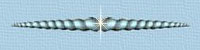﻿ Math Trick

# Math TrickI wonder how long someone worked on this to figure it out.
For all you mathematically challenged people, you may use the calculator below.

Enter the number of the month you were born,
multiply by 4, add 13, multiply by 25, subtract 200,
add the day of the month on which you were born,
multiply by 2, subtract 40, multiply by 50,
add the last two digits of the year in which you were born, subtract 10,500.

0
 7 8 9 / 4 5 6 * 1 2 3 - 0 +/- . +
 C
 =